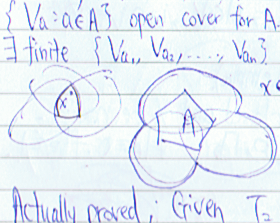Normal Compact $T_2$ Spaces

To show that any compact Hausdorff space is $T_4$, one may first show that it’s $T_3$. To see this, using set theory notations may be quite difficult. For mediocre students, the contents of the following seciton may sound unnatural.

A sketch of the proof

Suppose that we want to separate a point $x \notin A$ and a closed set $A$ in a compact $T_2$ space $X$ by two disjoint open sets $U$ and $V$ so that $x \in U$ and $A \subseteq V$. A standard proof is to apply the $T_2$ property of $X$ to $x$ and each $y \in A$ so as to yield two disjoint open sets $U_y,V_y \in \mathcal{T}$ such that $x \in U_y$ and $y \in V_y$. Since $A$ should be contained in an open set $V$, in other words, an open cover of $A$ is needed, one might be tempted to construct the following union of open sets.

\begin{equation} V = \bigcup_{y \in A} V_y \label{Vinf} \end{equation}

However, one can’t ensure that the following infinite intersection of open sets is open.

\begin{equation} U = \bigcap_{y \in A} U_y \label{Uinf} \end{equation}

For instance, by the Nested Interval Theorem,

$\{0\} = \bigcap_{n \in \N} \left( -\frac{1}{n},\frac{1}{n} \right).$

Thus, one applies the compactness of $X$ to get finite versions of \eqref{Vinf} and \eqref{Uinf}.

A snapshot of this fact

Since it’s so difficult to remember every detail of the proof, I love illustrating it using a picture.How can a compact regular space be regular? See my next post.